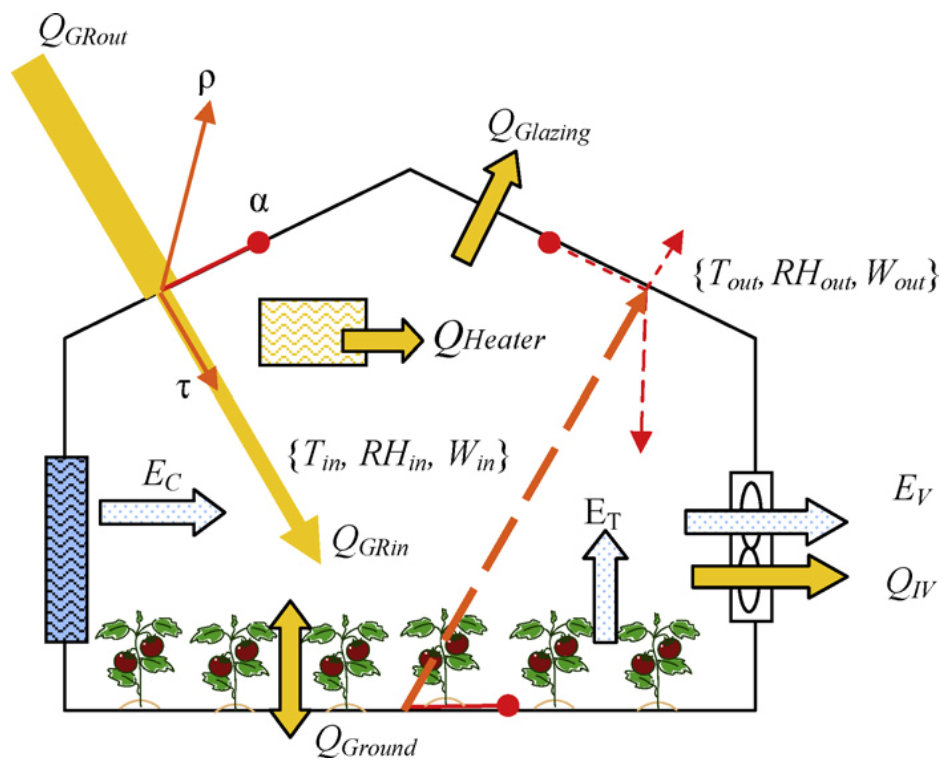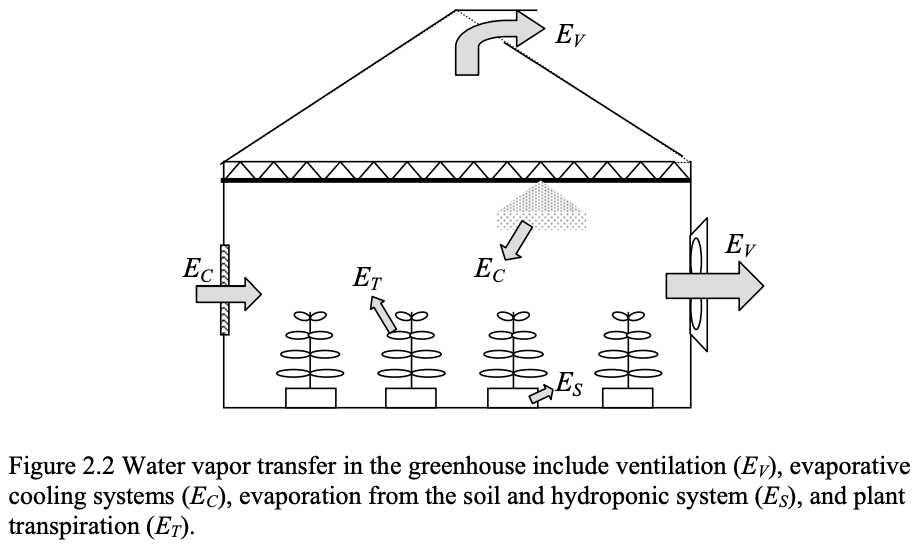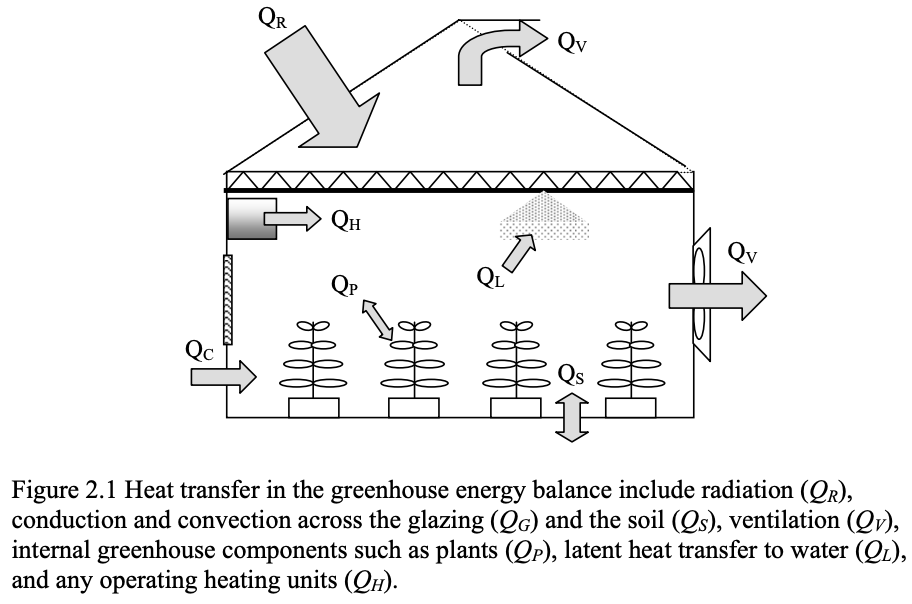Figure from Rodriguez et al. (2010).

## Water balance

A useful source is Nadia Christina Sabeh’s PhD thesis, 2007.$E_V + E_C + E_S + E_T = 0$

## Energy balance$Q_R + Q_G + Q_V + Q_S + Q_P + Q_L + Q_H = 0$

## Evaporative Cooling## Potential Evapotranspiration

### Penman (1948)

This equation accounts for two main terms:

• the first is the available energy flux density,
• the second term is the drying power of the air.
$PE = \frac{\Delta(R_n-G) + \gamma\cdot 2.6 (1+0.536 u_2)\text{VPD}}{\lambda(\Delta+\gamma)}$

### PyET

Luckily, we don’t have to work very hard to estimate the potential evapotranspiration. We will use the Python library pyet:

Using PyET is quite straightforward:

pyet.pm_fao56(tmean,               # mean temperature
wind,                # wind speed
rn=rn,               # net radiation
tmax=tmax,           # max temperature
tmin=tmin,           # max temperature
rh=rh,               # relative humidity
elevation=elevation) # elevation above sea level